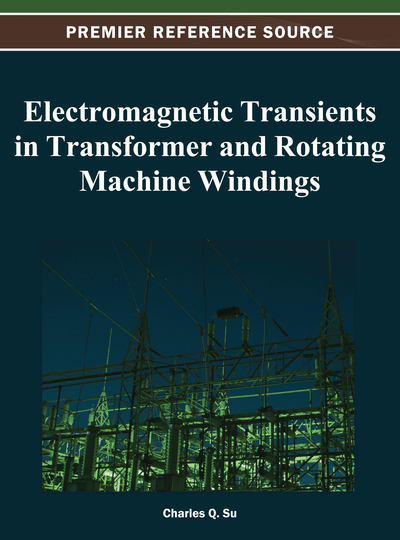# Transmission Line Theories for the Analysis of Electromagnetic Transients in Coil Windings

Akihiro Ametani, Teruo Ohno
DOI: 10.4018/978-1-4666-1921-0.ch001
OnDemand:
(Individual Chapters)
Available
\$29.50
No Current Special Offers

## Abstract

The chapter contains the basic theory of a distributed-parameter circuit for a single overhead conductor and for a multi-conductor system, which corresponds to a three-phase transmission line and a transformer winding. Starting from a partial differential equation of a single conductor, solutions of a voltage and a current on the conductor are derived as a function of the distance from the sending end. The characteristics of the voltage and the current are explained, and the propagation constant (attenuation and propagation velocity) and the characteristic impedance are described. For a multi-conductor system, a modal theory is introduced, and it is shown that the multi-conductor system is handled as a combination of independent single conductors. Finally, a modeling method of a coil is explained by applying the theories described in the chapter.
Chapter Preview
Top

## Introduction

When investigating transient and high-frequency steady-state phenomena, all the conductors such as a transmission line, a machine winding, and a measuring wire show a distributed-parameter nature. Well-known lumped-parameter circuits are an approximation of a distributed-parameter circuit to discuss a low-frequency steady-state phenomenon of the conductor. That is, a current in a conductor, even with very short length, needs a time to travel from its sending end to the remote end because of a finite propagation velocity of the current (300 m/μs in a free space). From this fact, it should be clear that a differential equation expressing the behavior of a current and a voltage along the conductor involves variables of distance x and time t or frequency f. Thus, it becomes a partial differential equation. On the contrary, a lumped-parameter circuit is expressed by an ordinary differential equation since there exists no concept of the length or the traveling time. The above is the most significant differences between the distributed-parameter circuit and the lumped-parameter circuit.

In this chapter, a basic theory of a distributed-parameter circuit is explained starting from impedance and admittance formulas of an overhead conductor. Then, a partial differential equation is derived to express the behavior of a current and a voltage in a single conductor by applying Kirchhoff’s law based on a lumped-parameter equivalence of the distributed-parameter line. The current and voltage solutions of the differential equation are derived by assuming (1) sinusoidal excitation and (2) a lossless conductor. From the solutions, the behaviors of the current and the voltage are discussed. For this, the definition and concept of a propagation constant (attenuation and propagation velocity) and a characteristic impedance are introduced.

As is well known, all the ac power systems are basically three-phase circuit. This fact makes a voltage, a current, and an impedance to be a three dimensional matrix form. A symmetrical component transformation (Fortesque and Clark transformation) is well-known to deal with the three-phase voltages and currents. However, the transformation cannot diagonalize an n by n impedance / admittance matrix. In general, a modal theory is necessary to deal with an untransposed transmission lines. In this chapter, the modal theory is explained. By adopting the modal theory, an n-phase line is analyzed as n-independent single conductors so that the basic theory of a single conductor can be applied.

In the last section of this chapter, the distributed-parameter theory is applied to model a coil winding. An example is demonstrated for a linear motor coil transient.

## Complete Chapter List

Search this Book:
Reset